Aegidius
Plüss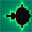www.aplu.ch      Print Text
 Home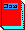Java exemplarisch (Textbook, in German)Programming Concepts in Python Tutorial and Exercises in German, French and English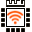LinkUp A WLAN Coprocessor Addon for the Arduino or Microbit (Released now)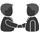TCPCom An event-driven socket libarry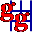JGameGrid        (Java Game Framework)JCardGame (JGameGrid Card Game Addon) Ported to AndroidJDroidLib     NEW: Support for Smartphone IDE (JGameGrid/TcpJLib for Android)JTurtleLib     (Java Turtle Graphics for Android)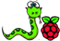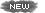Python-Exemplary Raspberry Pi Tutorial (in English and German)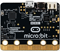micro:bit     Single-board Computer Python Tutorial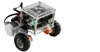BrickPiLib Library for the BrickPi Kit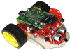RaspiBrick Low-Budget Robotics with the Raspberry Pi EV3JLib (Library for Lego EV3 Robot) Support for Jython & Android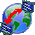TcpJLib (TCP/IP Communication)KinectJLib (Kinect Controller Library for Java)JAW       (Java API Wrapper, 32/64bit)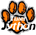Python/Jython (Using TigerJython IDE)Java Utilities  (Graphics, Turtle)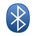Bluetooth Lib (For J2ME/J2SE)NxtJLib (Library for Lego NXT Robot) Support for J2SE/J2ME & AndroidXboxController (Xbox Gamepad Library)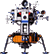Simulations    (for Physics Teachers)Overtone           (Spectral Composition/Analysis)FtController  (Fischertechnik Java Library) CoverSheet      (Presentation Tool) Gidlet      (Java ME Framework) MidPak      (MIDlet Packaging) JavaOC      (Java Object Creator) JawGadget (Transparent Windows) C++/Champ      (Graphics Framework) Home
GidletFirst example: Simple input and output

Purpose: Display an input form where the user enters a number (long). If an illegal character is typed, a warning beep is emitted and the character is erased. When the OK button is hit, the prime factors are calculated and displayed on an output form. When the OK button is hit there, the input dialog is shown again until the Exit button is hit.

// InOutGidlet.java
import
ch.aplu.gidlet.*;

public
class InOutGidlet extends Gidlet
{

public void main()

{

while (true)

{

long n = readLong("Enter a number:");

showMessage(n + " = " + getPrimeFactors(n, ""));

}

}

private String getPrimeFactors(long n, String result)

{

long divisor = primeFactor(n);

if (divisor == 0)

return result + n;

return getPrimeFactors(n / divisor, result + divisor + " x ");

}

private long primeFactor(long n)

{

for (long i = 2; i * i <= n; i++)

if (n % i == 0)

return i;

return 0;  // n is prime

}
}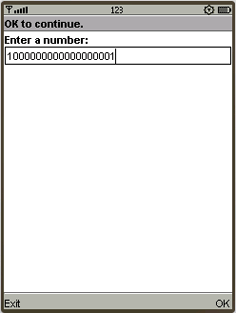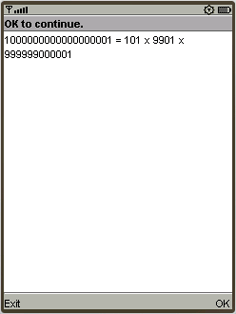Execute InOutGidlet (if you have the Sun's Wireless Toolkit (WTK) installed and the JAD extension registered. Learn how to register the JAD extension)

Discussion: A simple non-optimized recursive algorithm is used. For a given number n the method primeFactor() determines the first integer divisor d by trying to divide from 2 to the square root of n. The process is repeated for n / d until this is a prime number. Each time a divisor is found, it is appended to a result string (not very efficient, but simple).

Second example: Output in a console window

Purpose: Demonstrate that the sequence a(n) = (1 + 1/n)^n approaches e by writing a(n) for n = 1..100 in a console display.

 // LimitGidlet.java import ch.aplu.gidlet.*; public class LimitGidlet extends Gidlet {   public void main()   {     MConsole c = new MConsole();     int n = 1;     while (true)     {       c.print("a(" +  n + ") = ");       c.println(a(n));       waitOk();       n++;     }   }   private double a(double n)   {     return Float11.pow(1+1/n, n);   } }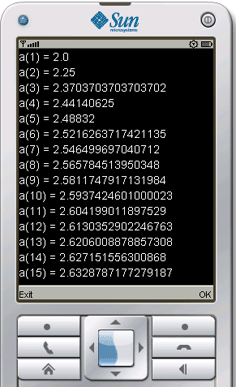Execute LimitGidlet (if you have the Sun's Wireless Toolkit (WTK) installed and the JAD extension registered. Learn how to register the JAD extension)

Discussion: We use the static Float11 method pow(x, y) to calculate x^y. In order to observe the convergence smoothly, the program is executed step-by-step by clicking the OK button. Even during the running program, the cursor up/down/left/right buttons may be used to scroll the display in the four directions.

Third example: Graphics with MPanel

Purpose: Same as example 2, but apply the proverb A Picture Worth A Thousand Words (numbers).

 // GraphLimitGidlet.java import ch.aplu.gidlet.*; public class GraphLimitGidlet extends Gidlet {   private MPanel p = new MPanel();   private String oldLabel = "";   public void main()   {     p.window(0, 50, 0, 3);     p.rectangle(0, 0, 50, 3);     for (int n = 1; n <= 50; n++)     {       show(n, a(n));       if (n == 1)         p.move(n, a(n));       else         p.draw(n, a(n));       waitOk();     }     p.color(RED);     p.line(0, Math.E, 50, Math.E);   }   private double a(double n)   {     return Float11.pow(1 + 1/n, n);   }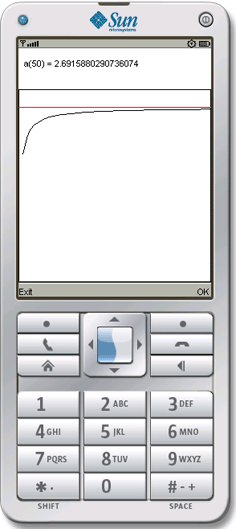private void show(int n, double a)   {     String newLabel = "  a(" + n + ") = " + a;     p.color(WHITE);     p.label(oldLabel, 3.5); // Erase     p.color(BLACK);     p.label(newLabel, 3.5); // Draw     oldLabel = newLabel;   } }

Execute GraphLimitGidlet (if you have the Sun's Wireless Toolkit (WTK) installed and the JAD extension registered. Learn how to register the JAD extension)

Discussion: We use the MPanel class and are happy to forget all about device dependant pixel coordinates by defining an application specific coordinate system. Drawing text into a graphics windows is somewhat cumbersome, because we must erase the old text by painting it in white color.Example Page 1 of 6 Example Page 2 of 6 Example Page 3 of 6 Example Page 4 of 6 Example Page 5 of 6 (MFile) Example Page 6 of 6 (StringStore) NxtJLibMP (Mobile Phone Addon for the Lego NXT Robot) Free Download Complete versionWMA2.0 compatible V 1.19- May 10 Free Download Downgraded versionWMA1.1 compatible V 1.19- May 10 FAQ/Forum Installation(general) Installation (using NetBeans) Gidlet mit Netbeans (in German) Online Learning (in German) ch.aplu.gidlet JavaDoc Gidlet Home